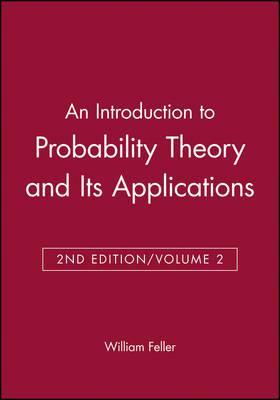# Why does mathematics model the universe, not recreates it.

in MES Sciencelast yearIn the introduction to the first volume of book of William Feller, there is an interesting philosophical part.
Think about such example:
Probabilist has to model the length of human life. Thus, for every nonnegativenumber x we want to know the probability, that human living in specific country will live longer than x years.
There are 2 possibilities, which are both counter-intuitive:

• if we create a continuous model, there would be nonnegative probability to survive ANY number of years. Probability that human will live 100000000 years will be like 0.0000000000000000000000000000000000000000000000000000000000000000000000000000000000000000000000000000000000000000000000000001%, but will be still greater than 0, which is totally counterintuitive;
• otherwise, if we create discrete model, then there would be a maximal length of human live. Assume that human can live at most 140 years. But if he can live 140 years, then he can easily live 140 years and 1 second. If he can live 140 years and one second, he can easily live 140 years and 2 seconds. And so on. Therefore, maximal possible time of live is also totally nonsense.
Both models are unreal, but we have to create model which is one of those two types.
This is great example that mathematics models universe, but does not recreates it.